Contents >> Engineering Mathematics >> Control Systems >> Dynamic Synthesis of Control System of Hydraulic Drive >> Appendix: Assessment of influence of variable elasticity, losses of pressure and leakages on natural frequency of hydraulic system

 Control Systems - Dynamic Synthesis - Appendix Appendix: Assessment of influence of variable elasticity, losses of pressure on length of pipelines and in local resistances and leakages on natural frequency of hydraulic system Let's consider the specific rated diagram of progressive hydraulic drive with the closed circulation of a stream (Fig. 5) of the section "Method of multiple periods of natural vibrations. Example" . On the basis of dependences (6) and (7) from the section "Method of multiple periods of natural vibrations. Algorithm":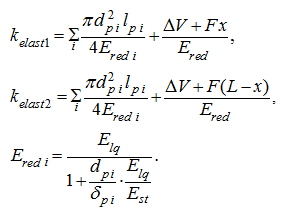factors of elasticity of cavities of the hydraulic system at its given parameters (see tab. 1 of the section "Method of multiple periods of natural vibrations. Example") are equal: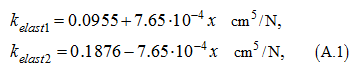where х – movement of the hydraulic cylinder piston in cm. Then the function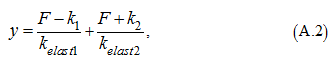entering into the radicand of (1) of the section "Method of multiple periods of natural vibrations. Example" for definition of natural frequency, at the given parameters of hydraulic system looks like: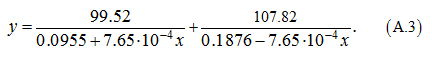At 0 ≤ х ≤ 120 (the full stroke of the hydraulic cylinder piston) у changes within the limits of: 14.7 ≤ у ≤ 16.65, that is the maximal change of function у at full stroke of the hydraulic cylinder piston will make: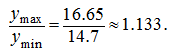At the same time at 0 ≤ х ≤ 64 (the given point of positioning) 14.7 ≤ у ≤ 16.3, that is change of у will make ~ 1.107. Thus, elasticity of the hydraulic system changes on ~ 11...13 %, consequently, change of natural frequency lays within the limits of ~ 5...6%. If to be limited to consideration of a range of variation of х , appropriating only to dispersal or braking, then influence on variation of natural frequency of the hydraulic system will appear even less and will make 3...4%. Thus, an existing inconstancy of elasticity of the hydraulic system renders very insignificant influence on value of natural frequency of the hydraulic drive. Let's consider the equations of dynamics of the hydraulic system presented on Fig. 5 of the section "Method of multiple periods of natural vibrations. Example" , at dispersal in view of leakages and losses of pressure on length of pipelines and in local resistances: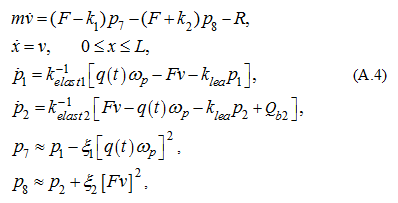where ξ 1 , ξ 2 – the reduced factors of total hydraulic losses on length of pipelines and in local resistances; р 7 , р 8 – pressures accordingly in pressure head and drain cavities of the hydraulic cylinder; k lea – factor of leakages of working liquid; Q b 2 – the boost flow defined by the static characteristic (3) of the section "Method of multiple periods of natural vibrations. Example" . The function v 2 we'll linearize, decomposing it in the power series in the neighborhood of v 0 and keeping only linear members of decomposition: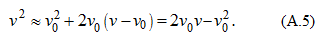Let's admit as v 0 an "average" value of speed: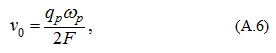then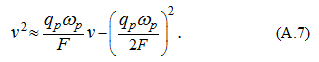In view of (3) from the section "Method of multiple periods of natural vibrations. Example" and (П.7) the characteristic equation of the system (П.4) looks like: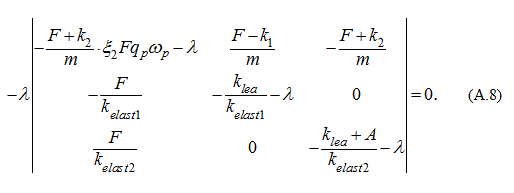According to the given numerical values of parameters (see tab. 1 of the section "Method of multiple periods of natural vibrations. Example") we'll receive: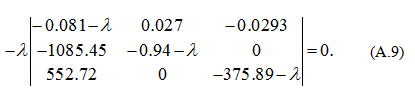Here A = 70.4 cm 5 /(N·s), ξ 1 = ξ 2 = 8·10 -6 N· s 2 /cm 8 . Expanding the determinant in the equation (П.9), we'll receive the following characteristic equation: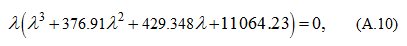which decision: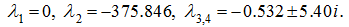Consequently, natural frequency of the hydraulic system is equal: а = 5.40 rad/s ≈ 0.859 Hz. From comparison of the received value of natural frequency and its value for the 3-rd rated version (~5.42 rad/s) is visible, that the account of losses of pressure on length of pipelines and in local resistances, as well as the account of leakages of working liquid leads to very minor clarification of natural frequency (~0.4 %), but to the more appreciable correction to factor of damping (without taking into account the specified factors this factor is equal about 0.0215, and with their account ~0.532). Thus the logarithmic decrement of attenuation makes ~0.62.

 < Previous Contents Next >